# The Paragyrate Diminished Rhombicosidodecahedron

The paragyrate diminished rhombicosidodecahedron is the 77th Johnson solid, or J77 for short. It has 52 faces (1 decagon, 11 pentagons, 25 squares, and 15 triangles), 105 edges, and 55 vertices. It is obtained by cutting off a pentagonal cupola segment from the rhombicosidodecahedron and gyrating the opposite pentagonal cupola.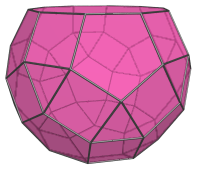The paragyrate diminished rhombicosidodecahedron closely resembles the diminished rhombicosidodecahedron (J76), but can be distinguished from the latter by 5 telltale pairs of adjacent squares, which are not present in J76: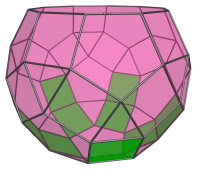These pairs of adjacent squares occur because of the gyrated orientation of the pentagonal cupola segment highlighted below, which lies antipodal to the decagonal face: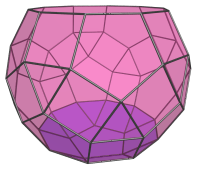## Projections

Here are some views of the paragyrate diminished rhombicosidodecahedron from various angles:

Projection Description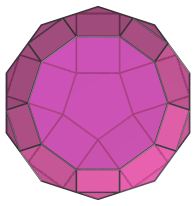Top view.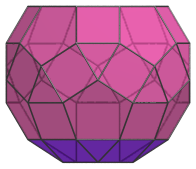Front view.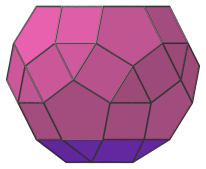Side view.

## Coordinates

The Cartesian coordinates of the paragyrate diminished rhombicosidodecahedron with edge length 2 are:

• (0, ±(2+φ), ±(1+φ))
• (±(2+φ), ±(1+φ), 0)
• ±(1+φ, 0, −(2+φ))
• (±1, ±1, ±(1+2φ))
• (±1, ±(1+2φ), ±1)
• ±(1+2φ, ±1, −1)
• (±φ, ±(1+φ), ±2φ)
• (±(1+φ), ±2φ, ±φ)
• ±(2φ, ±φ, −(1+φ))
• (−(8φ+1)/5, ±1, −(6φ+7)/5)
• (−(8φ+6)/5, ±φ, −(7+φ)/5)
• (−(13φ+1)/5, 0, −(φ+2)/5)

where φ=(1+√5)/2 is the Golden Ratio.

Last updated 07 May 2019.